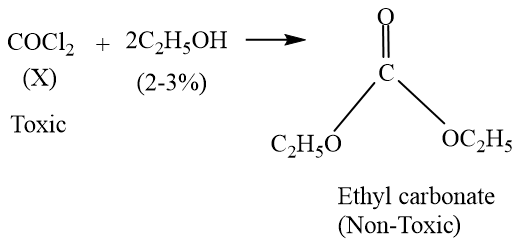Polyhalogen compounds
Question

# The reaction occuring when chloroform is exposed to sunlight and air is shown as ${\mathrm{CHCl}}_{3}\text{\hspace{0.17em}}+\text{\hspace{0.17em}}\frac{1}{2}\text{\hspace{0.17em}\hspace{0.17em}}{\mathrm{O}}_{2}\left(\mathrm{air}\right)\text{\hspace{0.17em}}\stackrel{\mathrm{Sunlight}}{\to }\text{\hspace{0.17em}\hspace{0.17em}}\mathrm{X}\text{\hspace{0.17em}\hspace{0.17em}}+\text{\hspace{0.17em}\hspace{0.17em}}\mathrm{HCl}.$    ‘X’ is toxic, it can be converted to a non toxic substance by the addition of 2-3% solution of

Moderate
Solution

##Get Instant Solutions